# Reinforcement Learning学习笔记（下）

## 入坑指南

Posted by HC on May 12, 2018

# 10. Model-based RL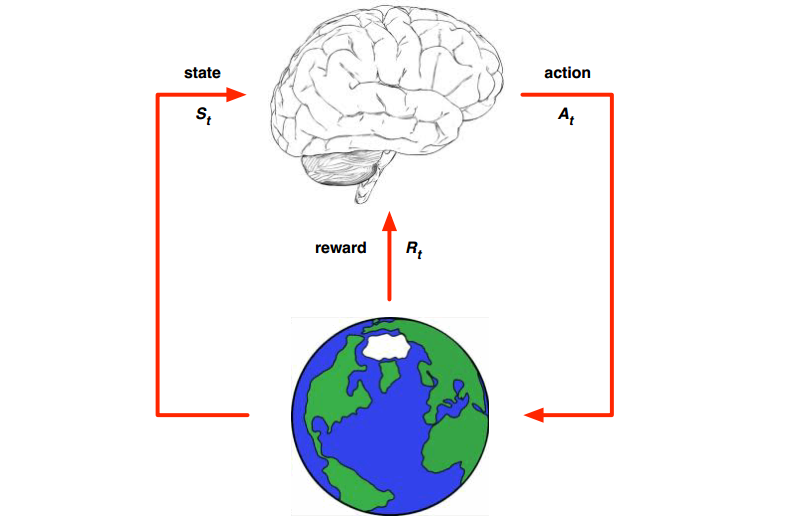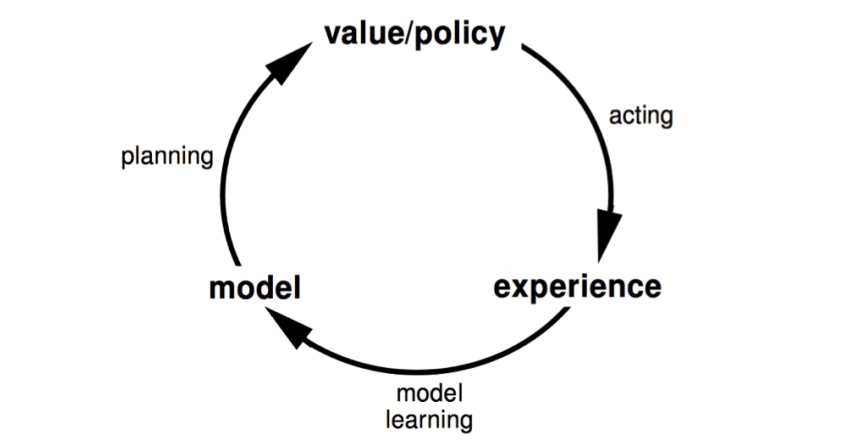## 10.1 Planning with learned dynamics

1. 用当前的policy/value，出招，得到一些反馈数据
2. 根据反馈数据，学习出一个dynamics $f$
3. 根据$f$来planning，优化policy/value

### 10.1.1 Distribution mismatch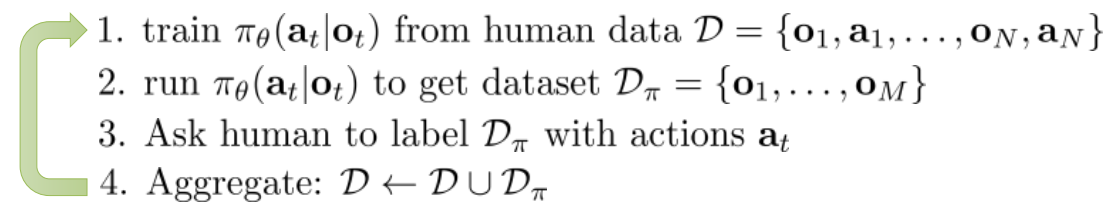### 10.1.2 Model error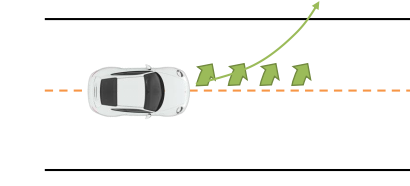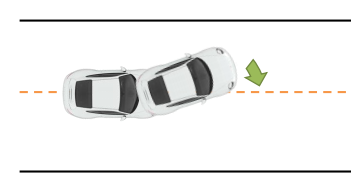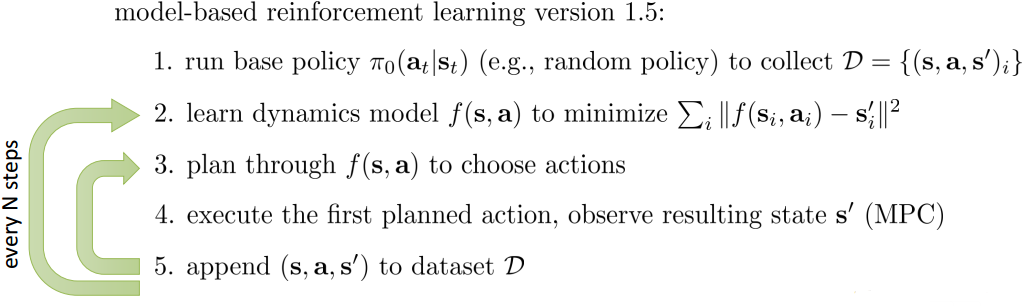## 10.2 Learn a model by supervised learning

$s_1,a_1 \rightarrow r_2,s_2\\ s_2,a_2 \rightarrow r_3,s_3\\ ...\\ s_{T-1},a_{T-1} \rightarrow r_{T},s_{T}\\$

1. Table Lookup Model
2. Linear Expectation model
3. linear gaussian
4. gaussian process
5. deep belief network
6. iLQR等等

### 10.2.1 Fitting global dynamic model

#### 10.2.1.1 Table Lookup Model

table lookup其实就是通过简单的数据统计，记录所有相同输入下的输出均值。可以将它视作一个全空间下的KNN回归模型，这个K等于数据点的个数。

$\widehat{P}_{ss'}^a=\frac{1}{N(s,a)}\sum_{t=1}^n1(S_t,A_t,S_{t+1}=s,a,s')\\ \widehat{R}_{s}^a=\frac{1}{N(s,a)}\sum_{t=1}^n1(S_t,A_t=s,a)R_t$

$A,0,B,0\\ B,1\\ B,1\\ B,1\\ B,1\\ B,1\\ B,1\\ B,0$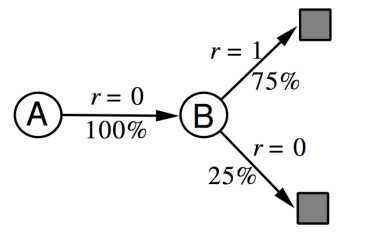$B,1\\ B,0\\ B,1\\ A,0,B,1\\ B,1\\ A,0,B,1\\ B,1\\ B,0$

#### 10.2.1.2 Dyna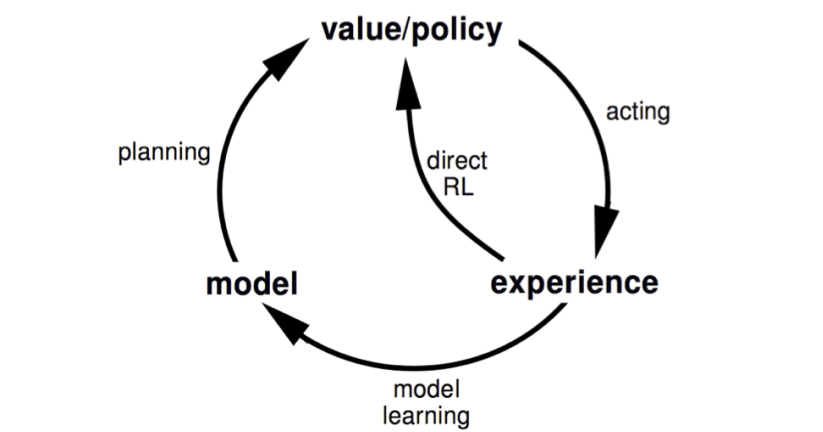Dyna如果建立上sample-based planning方法上，那么采样的数据将用于planning，这里planning选用的是model-free的q-learning，真实的数据也是通过Q-learning来更新value function。具体来说dyna的伪代码如下：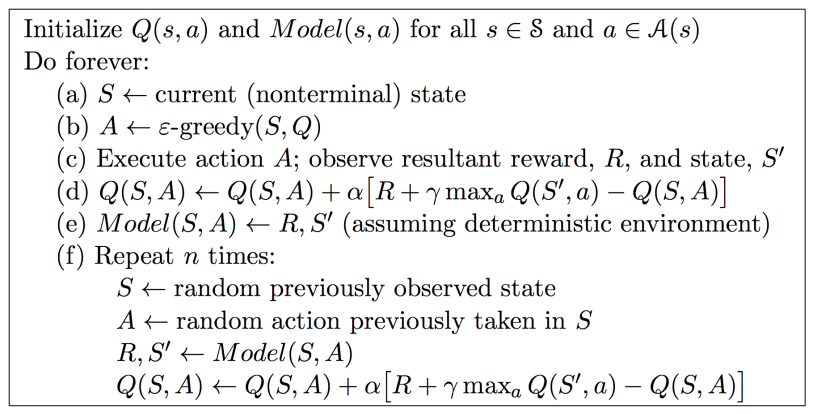### 10.2.2 Fitting local dynamic model

#### 10.2.2.1 Fit with iLQR

$\min_{a_1,... ,a_T} \sum_{t=1}^T c(s_t,a_t) \mbox{ s.t. } s_t=f(s_{t-1},a_{t-1})\\ \min_{a_1,... ,a_T} c(s_1,a_1) + c(f(s_1,a_1),a_2)+...+c(f(f(...)),a_T)$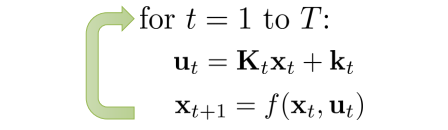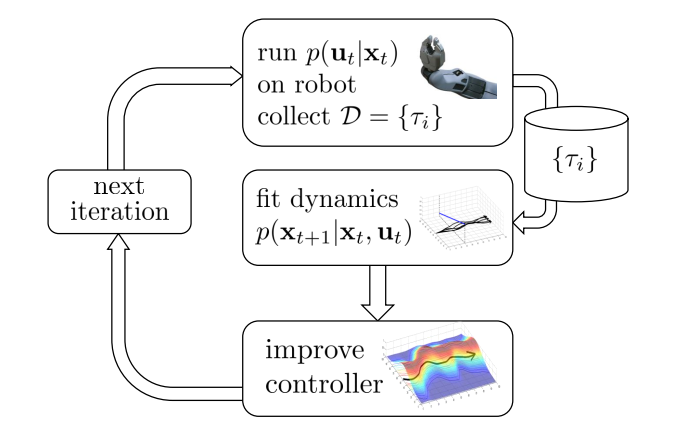1. 使用iLQR：
$p(a_{t}\mid s_{t})=\delta(a_{t}=K_{t}(s_{t}-\widehat{s}_{t})+k_{t}+\widehat{a}_{t})$
1. 考虑noise：
$p(a_{t}\mid s_{t})=N(K_{t}(s_{t}-\widehat{s}_{t})+k_{t}+\widehat{a}_{t}, \Sigma_{t})$

#### 10.2.2.2 Local model is too local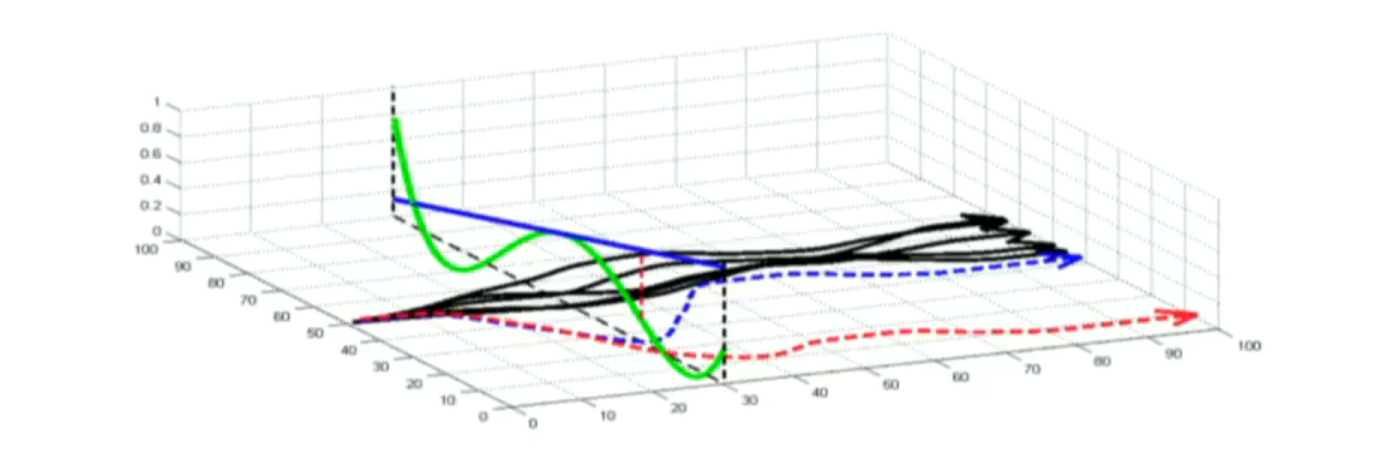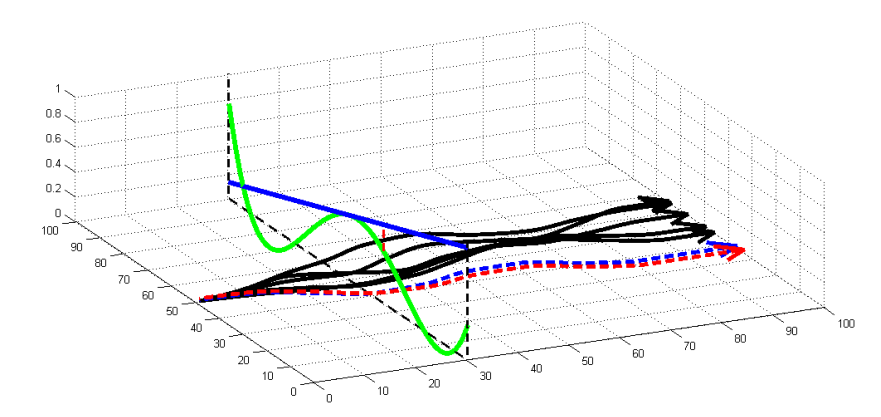$D_{KL}(P(\tau)\mid\mid \widehat{P}(\tau))=E_{P(\tau)}\left[ \sum_{t=1}^T logP(a_t\mid s_t) -log\widehat{P}(a_t\mid s_t)\right]\\ =\sum_{t=1}^TE_{p(s_t,a_t)} \left[ logP(a_t\mid s_t) -log\widehat{P}(a_t\mid s_t)\right]\\ =\sum_{t=1}^TE_{p(s_t,a_t)} \left[ -log\widehat{P}(a_t\mid s_t) - H(P(a_t\mid s_t))\right]$

$\min_P \sum_{t=1}^T E_{P(s_t,a_t)}[c(s_t,a_t)] \mbox{ s.t. } D_{KL}(P(\tau)\mid\mid \widehat{P}(\tau)) \leq \epsilon$$\min_P \sum_{t=1}^T E_{P(s_t,a_t)}[c(s_t,a_t) - \lambda log\widehat{P}(a_t\mid s_t) - \lambda H(P(a_t\mid s_t))] -\lambda \epsilon$

$\min_P \sum_{t=1}^T E_{P(s_t,a_t)}[c(s_t,a_t) - H(P(a_t\mid s_t))]$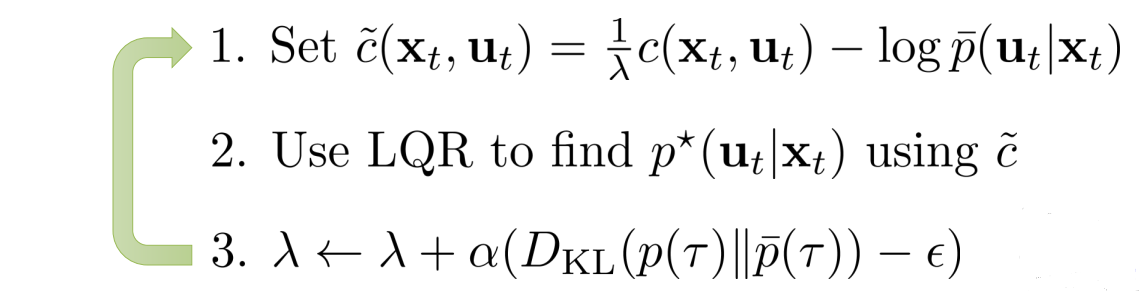## 10.3 Learn model and policy

model-based planning太慢（比如要做数据曾广，要做re-planning等等），难以给出实时响应，所以还是直接用policy比较好。比如打羽毛球时先根据环境和羽毛球的速度角度等等，planning一下落球点，然后跑过去接球。这个过程如果能得到一个好的接球policy的话，那么以后别人扔飞盘，就能不用重新planning一下，才能接了。所以policy隐含有更好的泛化能力。如果，我们能知道dynamics，那么整个序列决策过程可以用下图表示，那么就可以像deep model一样做back propagation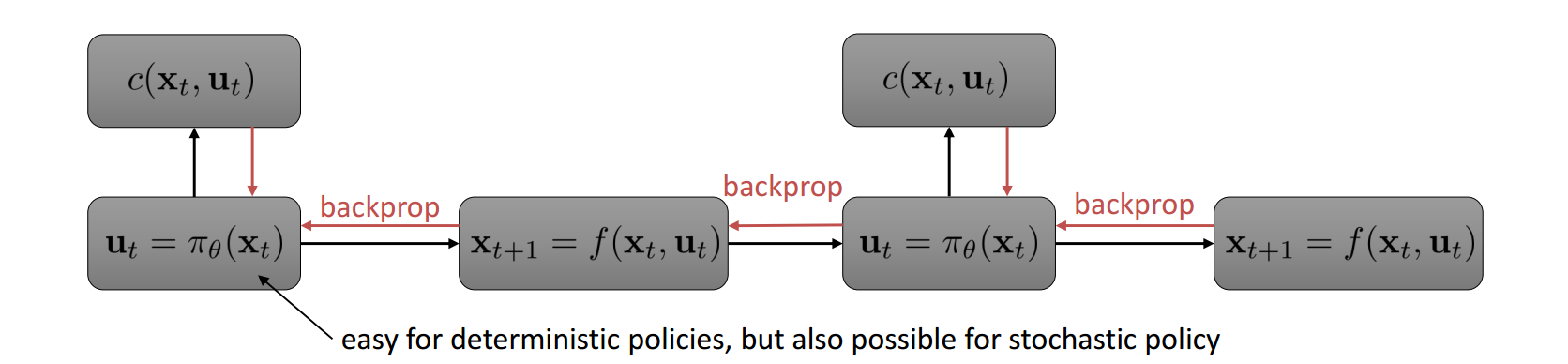$\min_{a_1,... ,a_T,s_1,...,s_T, \theta} \sum_{t=1}^T c(s_t,a_t) \mbox{ s.t. } s_t=f(s_{t-1},a_{t-1}), a_t=\pi_\theta(s_t)\\ \mbox{简化：}\min_{\tau, \theta} c(\tau) \mbox{ s.t. } a_t=\pi_\theta(s_t)$

$L(\tau,\theta,\lambda)=c(\tau)+\sum_{t=1}^T\lambda_t(\pi_\theta(s_t)-a_t) + \sum_{t=1}^T\delta_t(\pi_\theta(s_t)-a_t) ^2$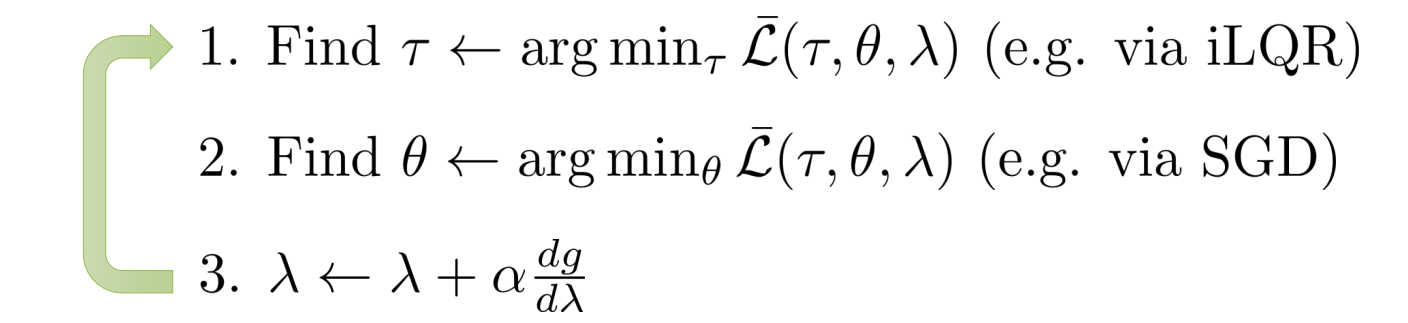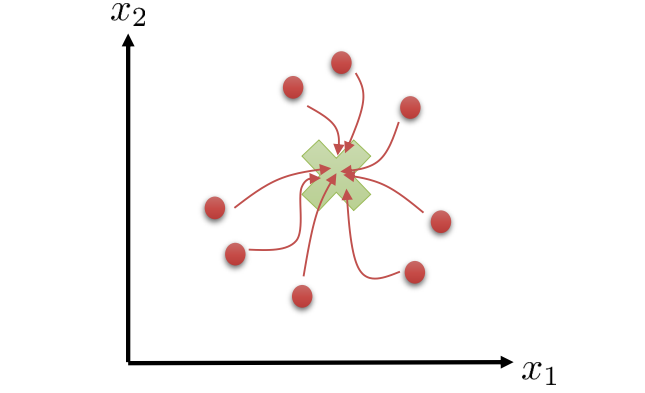$\min_{\tau_1,...,\tau_N, \theta} \sum_{i=1}^N c(\tau_i) \mbox{ s.t. } a_{t,i}=\pi_\theta(s_{t,i})$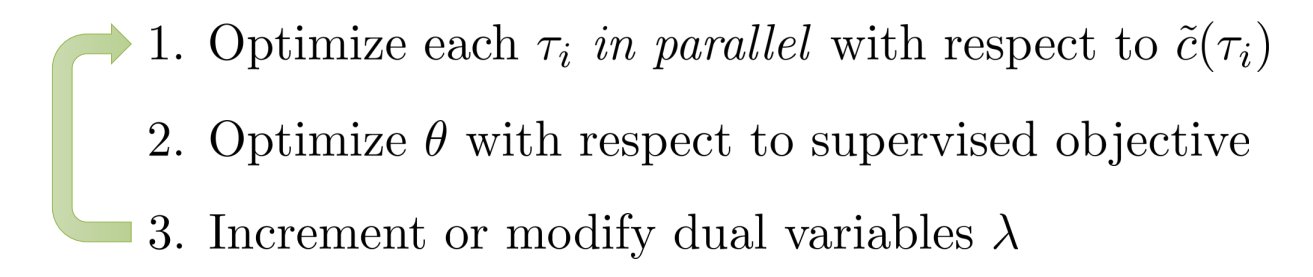# 11. Soft optimality, Inference and Control

$a_1,...,a_T=\arg\max_{a_1,...,a_T}\sum^T_{t=1} \gamma^{t-1}r(s_t,a_t) \mbox{ s.t. }s_{t+1}=f(s_t,a_t)\\ \pi=\arg\max E_{s_{t+1}\sim P(s_{t+1}\mid s_t,a_t),a_t\sim \pi(a_t \mid s_t)}[\gamma^{t-1}r(s_t,a_t)]$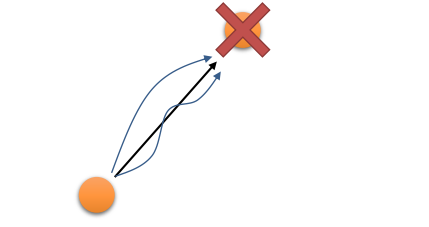## 11.1 Soft optimality and PGM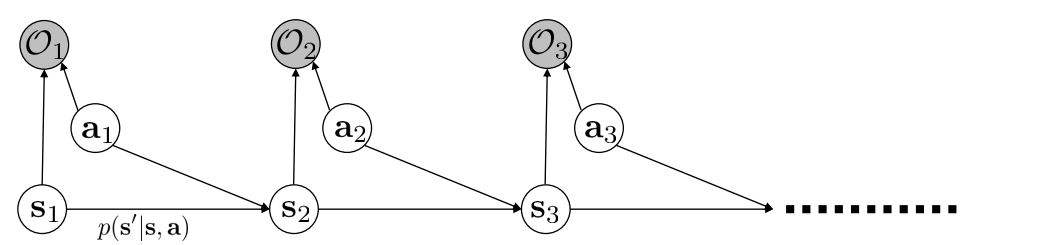PGM常常是用阴影的圆表示已观察值，空心表示未知变量（也就是state和action）。这里的变量$O$表示agent的goal或者intention。比如可以让$O$是0/1取值，表示猴子是不是想要接近目的地。是就$O_{t}=1$，否则是0。如此，我们便可以使用$P(\tau \mid O_{1:T})$表示在猴子为了要达到一定goal的条件下，这条trajectory的概率是多少。

$P(\tau \mid O_{1:T})=\frac{P(\tau)P(O_{1:T}\mid \tau)}{P(O_{1:T})} \propto P(\tau) \prod_t P(O_t \mid s_t,a_t)$

$P(\tau \mid O_{1:T}) \propto P(\tau) exp(\sum_t r(s_t,a_t))$

1. 计算backward message $\beta_{t}(s_{t},a_{t})=P(O_{t:T}\mid s_{t}, a_{t})$
2. 计算policy $P(a_{t} \mid s_{t}, O_{1:T})$
3. 计算forward message $\alpha_{t}(s_{t})=P(s_{t}\mid O_{1:t-1})$

## 11.2 Inference### 11.2.1 Backword message

$\beta_{t}(s_{t},a_{t})=P(O_{t:T}\mid s_{t}, a_{t})=P(O_t\mid s_t,a_t)P(O_{t+1:T}\mid s_t,a_t)$

$\beta_{t}(s_{t},a_{t})=P(O_{t:T}\mid s_{t}, a_{t})=\int P(O_{t:T}, s_{t+1}\mid s_{t}, a_{t}) ds_{t+1}\\ =\int P(O_t,O_{t+1:T}, s_{t+1}\mid s_{t}, a_{t}) ds_{t+1} \\ =\int P(O_{t+1:T}\mid s_{t+1})P(s_{t+1}\mid s_{t}, a_{t}) P(O_t\mid s_t,a_t) ds_{t+1}\\ =P(O_t\mid s_t,a_t)\int P(O_{t+1:T}\mid s_{t+1})P(s_{t+1}\mid s_{t}, a_{t}) ds_{t+1}$

$P(O_{t+1:T}\mid s_{t+1})=\int P(O_{t+1:T},a_{t+1}\mid s_{t+1}) da_{t+1}\\ =\int P(O_{t+1:T}\mid s_{t+1},a_{t+1})P(a_{t+1}\mid s_{t+1}) da_{t+1}$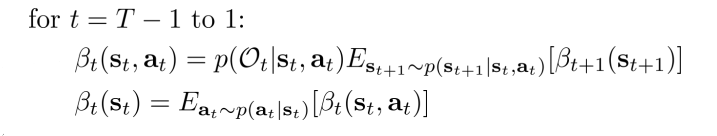$V_t(s_t)=\log \beta_t(s_t)\\ Q_t(s_t,a_t)=\log\beta_t(s_t,a_t)$

$V_t(s_t)=\log \int P(a_t \mid s_t) \exp(Q_t(s_t,a_t))da_{t}=\log \int \exp(\log P(a_t \mid s_t) + Q_t(s_t,a_t))da_{t}\\ Q_t(s_t,a_t)=r(s_t,a_t)+\log E_{s_{t+1} \sim P(s_{t+1}\mid s_t,a_t)}[exp(V_{t+1}(s_{t+1}))]$
1. 第一个式子的神奇之处就在于，他可以视作一个Soft max（不是神经网络里的softmax），是一种松弛的，用来求最大化的算子。在$Q_{t}(s_{t},a_{t})$变得很大的时候，$V_{t}(s_{t}) \rightarrow \max_{a_{t}}Q_{t}(s_{t},a_{t})$（注意，这里仍然假设$P(a_{t}\mid s_{t})$是uniform distribution。如果假设他是uniform的话，那么可以把$P(a_{t}\mid s_{t})$看做是reward的一部分：$\widehat{r}(s_{t},a_{t})=r(s_{t},a_{t})+\log P(a_{t}\mid s_{t})$）。

2. 对于第二个式子来说

1. 考虑deterministic transition的情况，就可以把期望用一个确定的转移状态替换：

$Q_t(s_t,a_t)=r(s_t,a_t)+V_{t+1}(s_{t+1})$
2. 有其他理论工作进一步可以将式子2改成下式：

$Q_t(s_t,a_t)=r(s_t,a_t)+E[V_{t+1}(s_{t+1})]$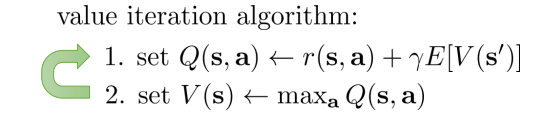$Q_t(s_t,a_t)=r(s_t,a_t)+\gamma E[V_{t+1}(s_{t+1})]$

$V_t(s_t)=\alpha \log \int \exp( \frac{\log P(a_t \mid s_t) + Q_t(s_t,a_t)}{\alpha})da_{t}$

### 11.2.2 policy

$\pi(a_{t} \mid s_{t})=P(a_{t} \mid s_{t}, O_{1:T})=\frac{P(a_t , s_t \mid O_{1:T})}{P(s_t\mid O_{1:T})}\\ =\frac{P(O_{1:T} \mid a_t,s_t)P(a_t,s_t)/P(O_{1:T})}{P( O_{1:T}\mid s_t)P(s_t)/P(O_{1:T})}\\ =\frac{\beta_t(s_t,a_t)}{\beta_t(s_t)}P(a_t|s_t)$

$\pi(a_{t} \mid s_{t})=\frac{\beta_t(s_t,a_t)}{\beta_t(s_t)}=\exp(Q_t(s_t,a_t)-V_t(s_t))=\exp(A_t(s_t,a_t))$

$\pi(a_{t} \mid s_{t})=\exp(\frac{Q_t(s_t,a_t)-V_t(s_t)}{\alpha})=\exp(A_t(s_t,a_t)/\alpha)$

### 11.2.3 forward mesage

$\alpha_{t}(s_{t})=P(s_{t}\mid O_{1:t-1})=\int P(s_t,s_{t-1},a_{t-1}\mid O_{1:t-1})ds_{t-1}da_{t-1}\\ =\int P(s_{t}\mid s_{t-1},a_{t-1})P(a_{t-1}\mid s_{t-1},O_{t-1})P(s_{t-1}\mid O_{1:t-2})ds_{t-1}da_{t-1}$

$P(a_{t-1}\mid s_{t-1},O_{t-1})=\frac{P(O_{t-1}\mid s_{t-1},a_{t-1})P(a_{t-1}\mid s_{t-1})}{P(O_{t-1} \mid s_{t-1})}$

$\alpha_{t}(s_{t})=P(s_{t}\mid O_{1:t-1}) \\ \propto \int P(s_{t}\mid s_{t-1},a_{t-1}) \exp(r(s_{t-1},a_{t-1}))\alpha_{t-1}(s_{t-1})ds_{t-1}da_{t-1}$

### 11.2.4 Inference in action

$P(s_{t}\mid O_{1:T})=\frac{P(s_t,O_{1:T})}{P(O_{1:T})}=\frac{P(O_{t:T}\mid s_{t})P(s_t,O_{1:t-1})}{P(O_{1:T})}\\ \propto \beta_t(s_t)\alpha_t(s_t) P(O_{1:t-1}) \propto \beta_t(s_t)\alpha_t(s_t)$

1. 前向信息$\alpha_{t}(s_{t})=P(s_{t}\mid O_{1:t-1})$表示观察到t-1时刻之前的intention，能到达到$s_{t}$状态的概率是多少（intention观察到越多，也就下一时刻的状态能被推测出的概率也就越大（前提是为了要接近目的地而设置的reward）。图右端大，左边窄）。
2. 后向信息$\beta_{t}(s_{t},a_{t})=P(O_{t:T}\mid s_{t}, a_{t})$表示从给定时刻t开始，完成goal的概率是多少（图左端大，右边窄）。
3. 所以从两个的定义来看，给定整个goal序列，那么某个时刻t，处于某一个状态的概率的大小就等价于前后向信息的乘积（从1到达t，以及从t到达T的概率乘积）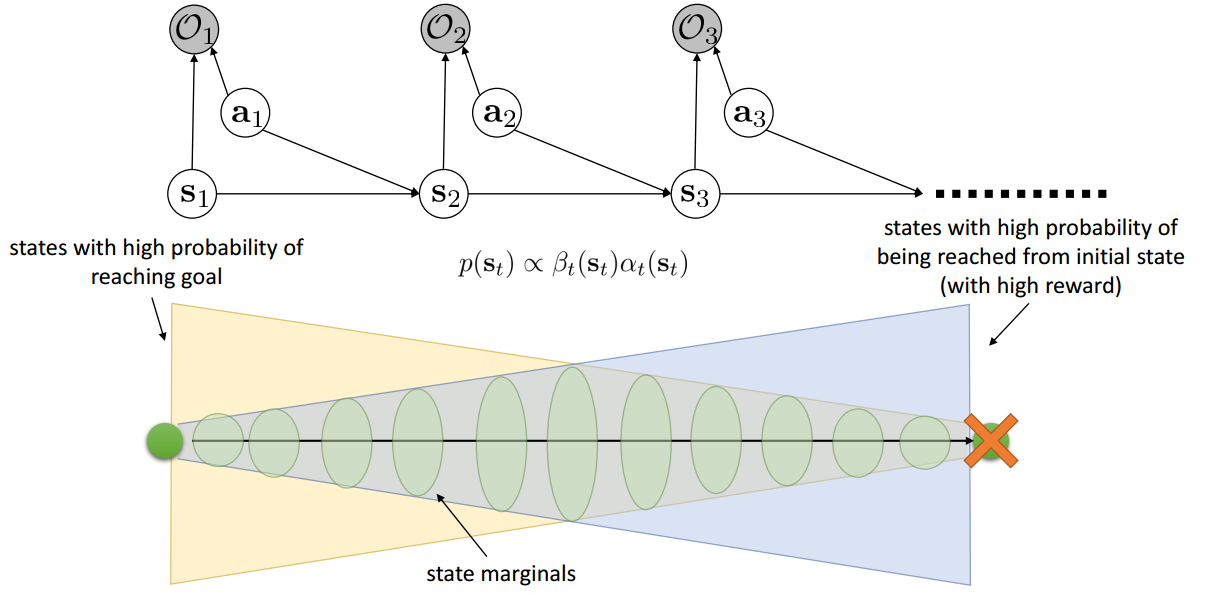## 11.3 Together with soft optimality

### 11.3.1 Q-learning with soft optimality

Q-learning是一个model-free off-policy的方法，他通过直接最大化Q来贪心的优化policy。这里off-policy是指采样数据不是来自policy本身，而是来自$\max_{a} Q(s,a)$。假设我们仍然采用function approximation，并且和target之间的loss选择L2，那么Q-learning的更新式子为：

$\phi \leftarrow \phi + \alpha \triangledown_\phi Q_\phi(s,a) \left( r(s,a) + \gamma V(s')- Q_\phi(s,a) \right)$

$V(s')=\log \int \exp(Q_\phi(s',a'))da'\\ \pi(a\mid s)=\exp(A(s,a))$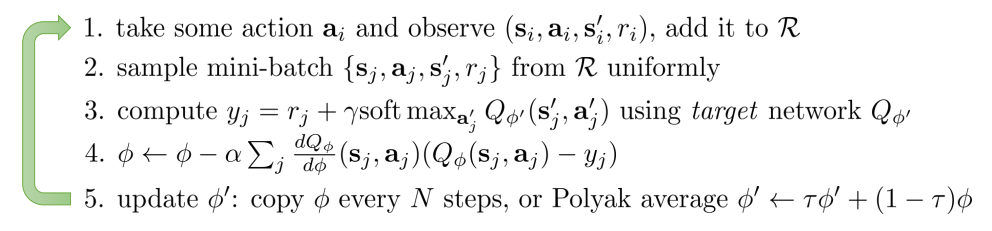### 11.3.2 Policy gradent with soft optimality

$\max \sum_t E_{\pi(s_t,a_t)} [r(s_t,a_t)] + E_{\pi(s_t)}[H(\pi(a_t\mid s_t))]=\sum_t E_{\pi(s_t,a_t)}[r(s_t,a_t)-\log \pi(a_t\mid s_t)]$

$\mbox{Soft policy gradient: }\frac{1}{N}\sum_t\sum_t\left( \triangledown_\theta Q(a_t\mid s_t) - \triangledown_\theta V(s_t)\right) \left( r(s_t,a_t) + Q(s_{t+1},a_{t+1})-Q(s_t,a_t)) \right)\\ \mbox{Soft Q-learning: }-\frac{1}{N}\sum_t\sum_t \triangledown_\theta Q(a_t\mid s_t) \left( r(s_t,a_t) + soft max Q(s_{t+1},a_{t+1})-Q(s_t,a_t)) \right)\\$
2. Q-learning的off-policy体现在soft max上。这里也可以使用on-policy的sarsa，这时候就没有soft max了，而是和policy gradent中的那一项一样

# 12. Inverse Reinforcement Learning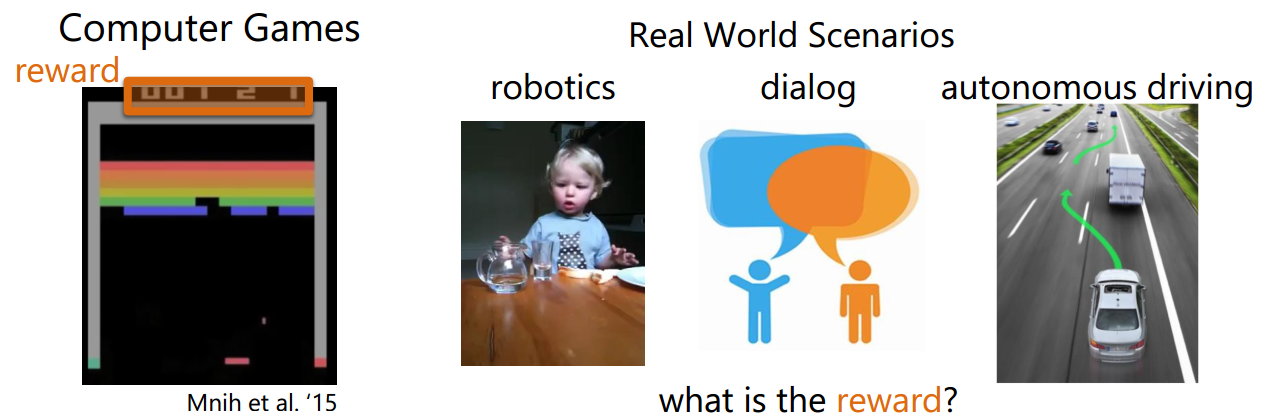IRL的目标就可以表示为，$\pi^\star \approx \pi^{r_{\psi}}$。也就说，要找一个reward function r，使得r下的最优policy $\pi^{r_\psi}$要尽可能的贴近在真实reward function下的最优策略$\pi^\star$。那么，下面朝着这个目标，我们来介绍一些IRL的算法

## 12.1 Feature matching based IRL

IRL的目标$\pi^\star \approx \pi^{r_{\psi}}$是很难直接刻画的，但是，如果两个policy一样，那么在相同的dynamics下，他们走出来的episode就应该很相似。Feature Matching就是从这个角度出发，它意思就说，要找一个参数$\psi$使得episode的特征的期望是相等的，即下式成立

$E_{\pi^{r_\psi}}[f(s,a)]=E_{\pi^\star}[f(s,a)]$

$\min \frac{1}{2} \mid \mid \psi \mid \mid^2\\ \mbox{s.t. } \psi^TE_{\pi^\star}[f(s,a)] \geq \max_{\pi} \psi^TE_{\pi}[f(s,a)] + D(\pi, \pi^\star)$

## 12.2 MaxEnt IRL

$P(\tau \mid O_{1:T}) \propto P(\tau) \exp\left( \sum_t r_\psi(s_t,a_t) \right)$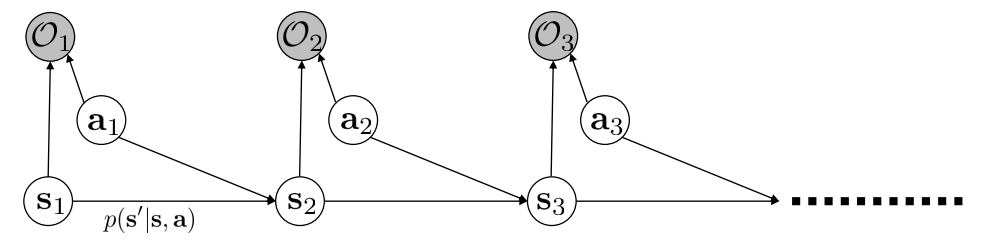$P(\tau \mid O_{1:T}, \psi)=\frac{P(\tau \mid O_{1:T}, \psi)}{\int P(\tau \mid O_{1:T}, \psi) d\tau}=\frac{P(\tau \mid O_{1:T}, \psi)}{Z}\\ \max_\psi \frac{1}{N} \sum_{i=1}^N \log P(\tau_i \mid O_{1:T}, \psi)=\frac{1}{N} \sum_{i=1}^N r_\psi(\tau_i) - \log Z$

$\triangledown_\psi L= \frac{1}{N}\sum_{t=1}^N \triangledown_\psi r_\psi(\tau_i) - \frac{1}{Z} \int P(\tau) \exp(r_\psi(\tau)) \triangledown_\psi r_\psi(\tau) d\tau\\ =E_{\tau \sim \pi^\star(\tau)} [\triangledown_\psi r_\psi(\tau)] - E_{\tau \sim P(\tau \mid O_{1:T}, \psi)}[\triangledown_\psi r_\psi(\tau)]$

$E_{\tau \sim P(\tau \mid O_{1:T}, \psi)}[\triangledown_\psi r_\psi(\tau)]= \sum_{t=1}^T E_{(s_t,a_t)\sim P(s_t,a_t \mid O_{1:T}, \psi) }[\triangledown_\psi r_\psi(s_t,a_t)]$

$P(s_t,a_t \mid O_{1:T}, \psi)=P(a_t\mid s_t,O_{1:T}, \psi) P(s_{t}\mid O_{1:T}, \psi)$

$P(a_t\mid s_t,O_{1:T}, \psi) = \frac{\beta(s_t,a_t)}{\beta(s_t)}\\ P(s_{t}\mid O_{1:T}, \psi) \propto \beta(s_t) \alpha(s_t)\\ P(s_t,a_t \mid O_{1:T}, \psi) \propto \beta(s_t,a_t)\alpha(s_t)$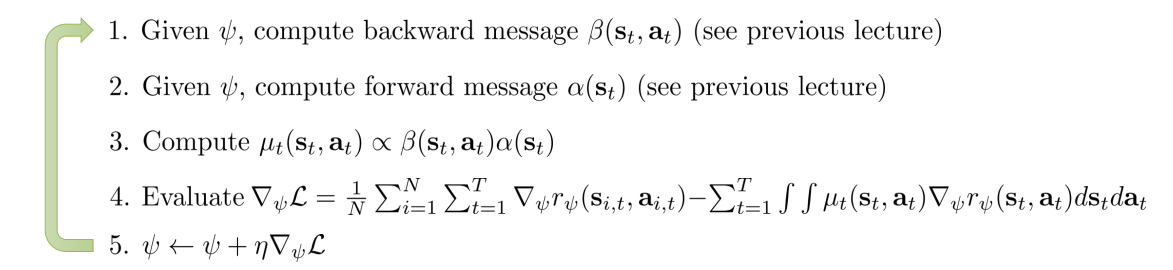$\max_\psi H(\pi^{r_\psi}) \\ \mbox{s.t. }E_{\pi^{r_\psi}}[f(s,a)]=E_{\pi^\star}[f(s,a)]$

## 12.3 Sampling-based MaxEnt IRL

$\triangledown_\psi L=E_{\tau \sim \pi^\star(\tau)} [\triangledown_\psi r_\psi(\tau)] - E_{\tau \sim P(\tau \mid O_{1:T}, \psi)}[\triangledown_\psi r_\psi(\tau)]\\ \approx \frac{1}{N} \sum^N_{t=1} \triangledown_\psi r_\psi(\tau_i) - \frac{1}{M} \sum^M_{j=1} \triangledown_\psi r_\psi(\tau_j)$

$\triangledown_\psi L \approx \frac{1}{N} \sum^N_{t=1} \triangledown_\psi r_\psi(\tau_i) - \frac{1}{\sum_j w_j} \sum^M_{j=1} w_j \triangledown_\psi r_\psi(\tau_j)\\ w_j = \frac{\pi^{r_\psi}(\tau_j)}{\pi^{IS}(\tau_j)}=\frac{P(\tau \mid O_{1:T})}{P^{IS}(\tau)}=\frac{P(s_1)\prod_t P(s_{t+1}\mid s_t)P(a_t\mid s_t)\exp(r_\psi(s_t,a_t))}{P(s_1)\prod_t P(s_{t+1}\mid s_t)\pi(a_t\mid s_t)}\\ =\frac{\prod_t P(a_t\mid s_t)\exp(r_\psi(s_t,a_t))}{\prod_t \pi(a_t\mid s_t)}$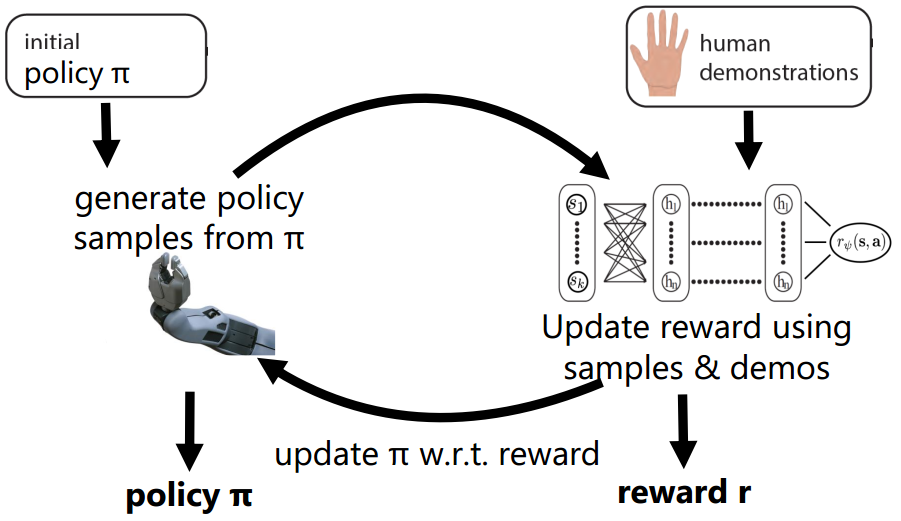## 12.4 GAN & IRL

[NIPS’16] Generative Adversarial Imitation Learning

[ICML’17] Use learned dynamics model to backdrop through discriminator

$\theta^*=\arg\max_\theta E_{\tau \sim \pi_\theta(\tau)} \left[ \sum_{t=1} \gamma^{t-1} r(s_t,a_t) \right]\\ \bigtriangledown_\theta J(\theta)=E_{\tau \sim \pi_\theta(\tau)} \left[ \left(\sum_{t=1}^T \bigtriangledown_\theta log \pi_\theta(a_t\mid s_t) \right) \left( \sum_{t=1}^T \gamma^{t-1} r(s_t,a_t)\right) \right]$

$\bigtriangledown_\theta J(\theta)=E_{\tau \sim \pi_\theta(\tau)} \left[ \sum_{t=1}^T \gamma^t \bigtriangledown_\theta log \pi_\theta(a_t\mid s_t) A^{\pi_\theta}(s_t,a_t) \right]$

$\bigtriangledown_\theta J(\theta) = E_{\tau \sim \pi'(\tau)} \left[\frac{\pi_\theta(\tau)}{\pi'(\tau)} \bigtriangledown_\theta log \pi_\theta(\tau) r(\tau)\right]$

但是Importance sampling的策略还是有两个bugs，第一，IS来带的方差是很大的！从图上来看，如果使用target是P，IS用Q来采样的话，在Q和P的相似性不高的时候，Q会采样出很多几乎无效的样本（他们的权重很小，图中的bar表示权重）。而且很难选中一个和P相似的Q。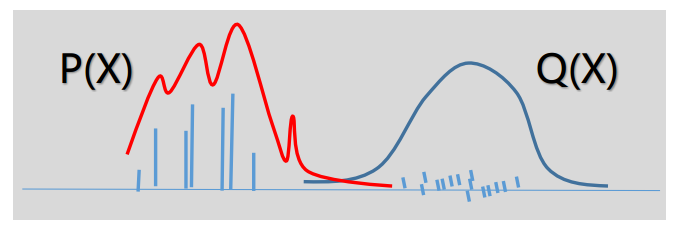从数学上来说：IS做的核心就是下式：

$E_{x \sim P} [ f(x)] = E_{x \sim Q}\left[ \frac{P(x)}{Q(x)}f(x) \right]$

但是，它的方差就很恐怖了

$Var\left(\frac{P(x)}{Q(x)}f(x)\right)=E_{x \sim Q} \left[ (\frac{P(x)}{Q(x)}f(x))^2 \right] - E_{x \sim Q} \left[ \frac{P(x)}{Q(x)}f(x) \right]^2\\ = \int Q(x) (\frac{P(x)}{Q(x)}f(x))^2 dx - (\int \frac{P(x)}{Q(x)}f(x) Q(x) dx)^2\\ =E_{x \sim P}\left[ \frac{P(x)}{Q(x)}f(x)^2 \right] - E_{x \sim P}\left[ f(x) \right]^2$

可以看到，一旦$\frac{P(x)}{Q(x)}$变大，那么这个方差是很大的。另外，IS的第二个bug在于Exploding or vanishing importance sampling weights。当使用IS的时候，policy gradient可以写成下式：

$\bigtriangledown_\theta J(\theta) =E_{\tau \sim \pi'(\tau)} \left[ \sum_{t=1}^T \bigtriangledown_\theta log \pi_\theta(a_{i,t}\mid s_{i,t}) \left(\prod_{t'=1}^t \frac{\pi_\theta(a_t'\mid s_t')}{\pi'(a_t'\mid s_t')}\right) \left( \sum_{t‘=t}^T \gamma^{t'-t} r(s_{i,t'},a_{i,t'})\right)\right]$

问题就出在式子中的叠乘项。即使如果一条episode中，每个时刻Q和P只有一点点不一样，但是他们叠乘起来，这个数就会变得很畸形了。更不要说还可能是多个小于1的数叠乘会引发weights vanishing，多个大于1的数叠乘会引发weight exploding。总的来说，求解效果会很不稳定。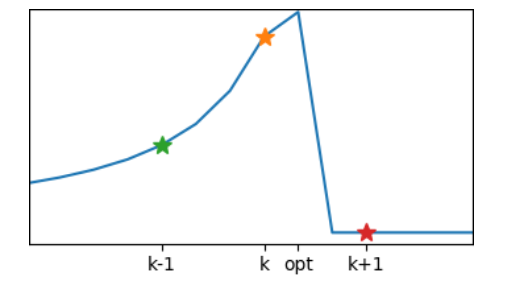3. 参数空间不等于policy 空间。感觉这算是一个收敛敏感性的问题。比如下图参数$\theta$发生等距的变化（从4, 再到2, 最后到0）但是在policy的变化却可能会很大。policy空间可能参数敏感的，在这种情况的优化policy，会很困难。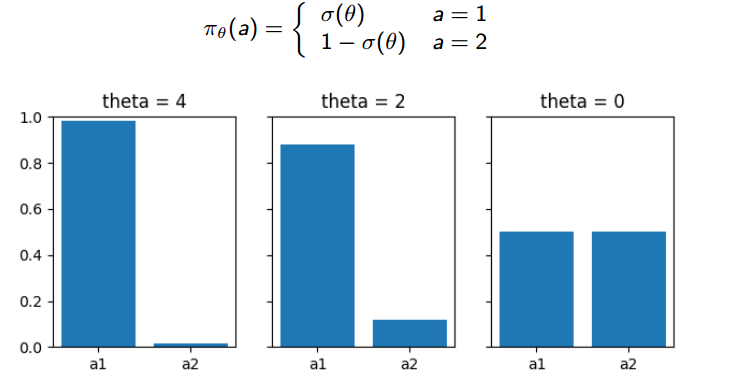另一个例子是下图。当均值都从-1变到1的时候，很明显第一个图中的Gaussian变化更大。所以，不同的参数对分布的影响是不同的（比如均值和方差），传统的梯度下降是将每个参数的影响都视为一样的，因此，我们需要将参数对整体输出的影响考虑进去，并且根据它来scale每一个参数。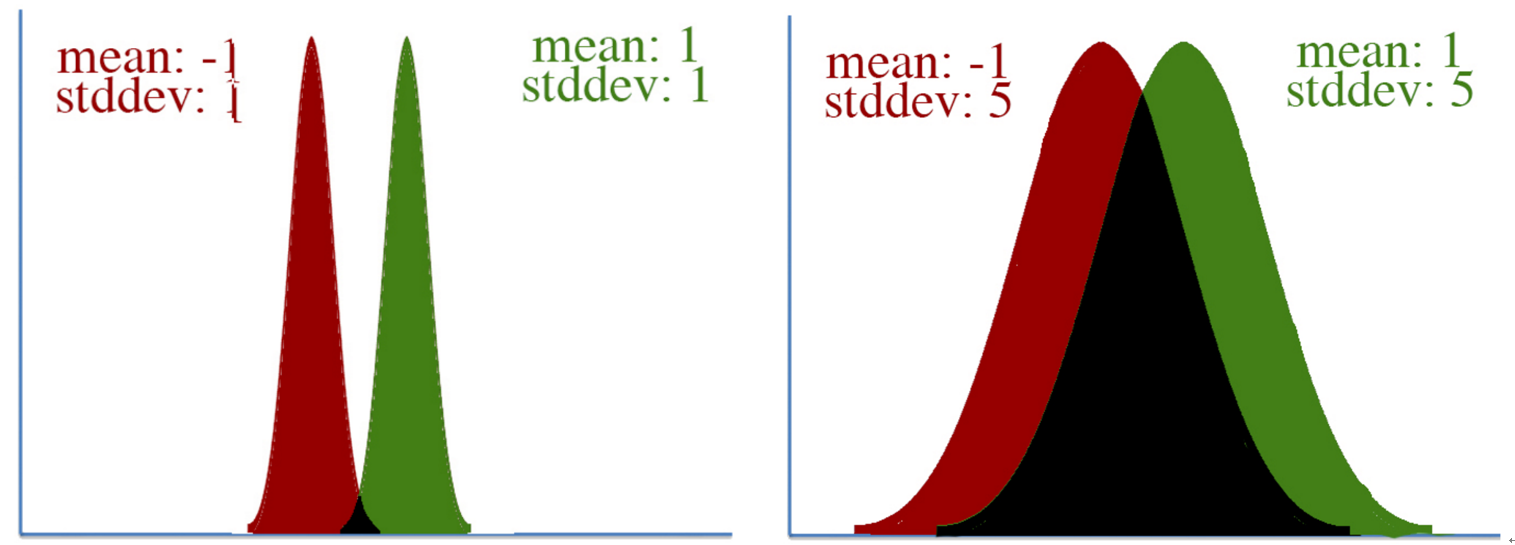## 13.1 Policy performance bounds

$J(\pi_\theta)=E_{\tau \sim \pi_\theta(\tau)} \left[ \sum_{t=1} \gamma^{t-1} r(s_t,a_t) \right]$

$J(\pi')-J(\pi)=E_{\tau \sim \pi'} \left[ \sum_{t=0} \gamma^t A^\pi(s_{t},a_{t})\right]$

$J(\pi')-J(\pi)=E_{\tau \sim \pi'} \left[ \sum_{t=0} \gamma^t A^\pi(s_{t},a_{t})\right]\\ =E_{\tau \sim \pi'} \left[ \sum_{t=0} \gamma^t (r(s_t,a_t)+\gamma V^\pi(s_{t+1}) - V^\pi(s_t))\right]\\ =J(\pi') + E_{\tau \sim \pi'} \left[ \sum_{t=0} \gamma^{t+1} V^\pi(s_{t+1}) - \sum_{t=0} \gamma^t V^\pi(s_t)\right]\\ =J(\pi') + E_{\tau \sim \pi'} \left[ \sum_{t=1} \gamma^{t} V^\pi(s_{t+1}) - \sum_{t=0} \gamma^t V^\pi(s_t)\right]\\ =J(\pi') + E_{\tau \sim \pi'}[V^\pi(s_0)] =J(\pi')-J(\pi)$

$\pi^{(k+1)}=\arg\max_{\pi'} J(\pi')-J(\pi^{(k)})=E_{\tau \sim \pi'} \left[ \sum_{t=0} \gamma^t A^{\pi^{(k)}}(s_{t},a_{t})\right]$

$d^\pi(s)=(1-\gamma) \sum_{t=0}^\infty \gamma^t P(s_t=s \mid \pi)$

$J(\pi')-J(\pi)=E_{\tau \sim \pi'} \left[ \sum_{t=0} \gamma^t A^\pi(s_{t},a_{t})\right]\\ =\sum_{t=0} \sum_{s}\gamma^t P(s_t=s\mid \pi') \sum_{a} \pi'(a\mid s) A^\pi(s_{t},a_{t})\\ =\frac{1}{1-\gamma} E_{s \sim d^{\pi'}, a \sim \pi'} [A^\pi(s,a)] \\ =\frac{1}{1-\gamma} E_{s \sim d^{\pi'}, a \sim \pi} [ \frac{\pi'(a \mid s)}{\pi(a\mid s)} A^\pi(s,a)]\\ \approx \frac{1}{1-\gamma} E_{s \sim d^{\pi}, a \sim \pi} [ \frac{\pi'(a \mid s)}{\pi(a\mid s)} A^\pi(s,a)] = L_\pi(\pi')\\ =E_{\tau \sim \pi} \left[ \sum_{t=0} \gamma^t \frac{\pi'(a_t \mid s_t)}{\pi(a_t\mid s_t)} A^\pi(s_t,a_t) \right]$

$\mid J(\pi')-(J(\pi)+L_\pi(\pi')) \mid \leq C \sqrt{E_{s \sim d^\pi}[D_{KL}(\pi' \mid \mid \pi)[s]]}\\ D_{KL}(\pi' \mid \mid \pi)[s]=\sum_{a} \pi'(a\mid s)\log \frac{\pi'(a\mid s)}{\pi(a\mid s)}$

$\triangledown_\theta L_{\theta_k}(\theta) \mid_{\theta_k} = E_{\tau \sim \pi_{\theta_k}} \left[ \sum_{t=0} \gamma^t \frac{\triangledown_\theta \pi_\theta(a_t \mid s_t) \mid _{\theta_k}}{\pi_{\theta_k}(a_t \mid s_t)} A^{\pi_{\theta_k}}(s_t,a_t) \right] \\ =E_{\tau \sim \pi_{\theta_k}} \left[ \sum_{t=0} \gamma^t \triangledown_\theta\log \pi_\theta(a_t\mid s_t) \mid_{\theta_k} A^{\pi_{\theta_k}}(s_t,a_t) \right]$

## 13.2 Monotonic Improvement Theory

$\pi^{(k+1)}=\arg\max_{\pi'} L_{\pi^{(k)}}(\pi' )- C \sqrt{E_{s \sim d^{\pi^{(k)}}}[D_{KL}(\pi' \mid \mid \pi^{(k)})[s]]}\\ \mbox{因为：} J(\pi') - J(\pi) \geq L_\pi(\pi') - C\sqrt{E_{s \sim d^{\pi^{(k)}}}[D_{KL}(\pi' \mid \mid \pi^{(k)})[s]]}$

$\pi^{(k+1)}=\arg\max_{\pi'} L_{\pi^{(k)}}(\pi' )\\ \mbox{s.t. }E_{s \sim d^{\pi^{(k)}}}[D_{KL}(\pi' \mid \mid \pi^{(k)})[s]] \leq \delta$

### 13.3.1 Natural Policy Gradient (NPG)

NPG主要是为了处理parameters space和policy space的in-covariant而提出的。他最后的下降方向是$H^{-1}g$，其中$g$是梯度，$H$是一个Fisher Information matrix。NPG通过使用H来scale parameter，达到covariant 的目的（也就是前文提到的PG的第三个问题）。当$H=I$的时候，NPG就是普通的PG下降方向了。

Under specific regularity conditions，fisher information刻画了最大似然估计的方差，等同于log 似然的负二阶导数的期望 $-E[\frac{\partial^2 \log L(X;\theta)}{\partial \theta^2}]$.。Fisher Information反映了我们对参数估计的准确度，它越大，对参数估计的准确度越高，即代表了越多的信息。

NPG优化细节是通过用泰勒展开来近似原始目标方程。

$\max_{\pi'} L_{\pi^{(k)}}(\pi' )\\ \mbox{s.t. }D_{KL}(\pi' \mid \mid \pi^{(k)})\leq \delta$

$L_{\theta_k}(\theta) \approx L_{\theta_k}(\theta_k) + g^T (\theta - \theta_k) , \mbox{ } g=\triangledown_\theta L_{\theta_k}(\theta) \mid_{\theta_k}\\ D_{KL}(\theta \mid \mid \theta_k) \approx \frac{1}{2} (\theta-\theta_k)^T H (\theta-\theta_k), \mbox{ } H= \triangledown^2_\theta D_{KL}(\theta \mid \mid \theta_k)\mid_{\theta_k}$

$D_{KL}(\theta \mid \mid \theta_k) \approx D_{KL}(\theta_k \mid \mid \theta_k) + g_{KL}^T (\theta-\theta_k)+ \frac{1}{2} (\theta-\theta_k)^T H (\theta-\theta_k)$

$D_{KL}(\theta \mid \mid \theta_k) = \int \pi_\theta(s) \frac{\log \pi_\theta(s)}{\log \pi_{\theta_k}(s)} ds\\ \triangledown_\theta D_{KL}(\theta \mid \mid \theta_k) = \int \triangledown [ \pi_\theta(s) \log \pi_\theta(s)] ds - \int \triangledown \pi_\theta(s) \log \pi_{\theta_k}(s) ds\\ = \int \triangledown \pi_\theta(s)(1+\log \pi_\theta(s) ) ds - \int \triangledown \pi_\theta(s) \log \pi_{\theta_k}(s) ds\\ = \int \triangledown \pi_\theta(s)(1+\log \pi_\theta(s) - \log \pi_{\theta_k}(s) )ds \\ = \int \triangledown \pi_\theta(s)(\log \pi_\theta(s) - \log \pi_{\theta_k}(s) )ds\\ \mbox{因此：}g_{KL}^T=\triangledown_\theta D_{KL}(\theta \mid \mid \theta_k)\mid_{\theta_k} =0$

$\int \triangledown \pi_\theta(s) ds = \triangledown \int \pi_\theta(s) ds = \triangledown 1 = 0$

$\theta_{k+1} = \arg \max_\theta g^T (\theta - \theta_k) \\ \mbox{s.t. } \frac{1}{2} (\theta - \theta_k)^T H (\theta - \theta_k) \leq \delta$

$L= g^T (\theta_k - \theta) - \lambda \left( \frac{1}{2} (\theta - \theta_k)^T H (\theta - \theta_k) - \delta \right)$

1. stationarity condition: $\frac{\partial L}{\partial \theta}=0 \rightarrow \theta=\theta_{k}+\frac{1}{\lambda} H^{-1}g$
2. complementary condition: $\lambda \left( \frac{1}{2} (\theta - \theta_{k})^T H (\theta - \theta_{k}) - \delta \right)=0$
3. primal & dual feasibility: 略

$\left\{\begin{matrix} \theta=\theta_{k}+\frac{1}{\lambda} H^{-1}g \\ (\theta - \theta_{k})^T H (\theta - \theta_{k}) = 2\delta \end{matrix}\right.$

$\theta=\theta_{k}+ \sqrt{\frac{2\delta}{g^TH^{-1}g}} H^{-1}g$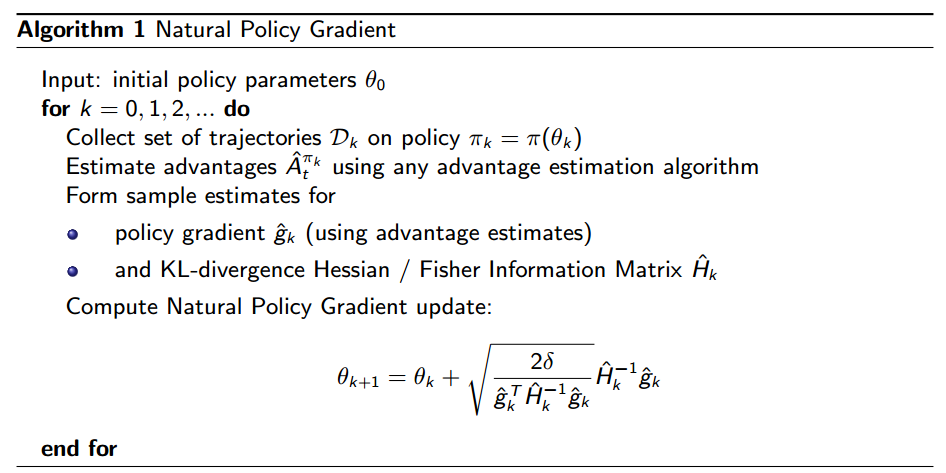### 13.3.2 Trust Region Policy Optimization (TRPO)

NPG算法很厉害，但是在实际使用中有两个问题：

1. $\delta$的选择
2. 泰勒展开近似KL散度的时候，原始约束可能会被破坏，不准确。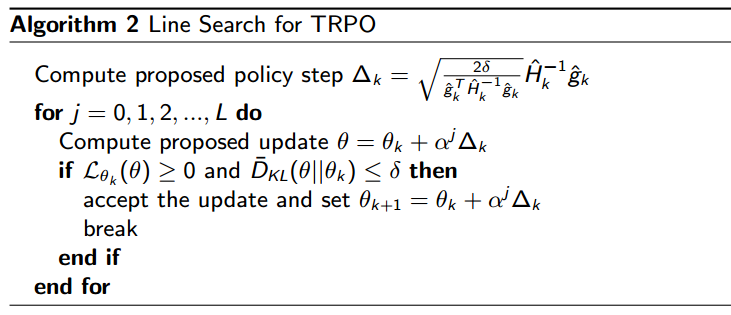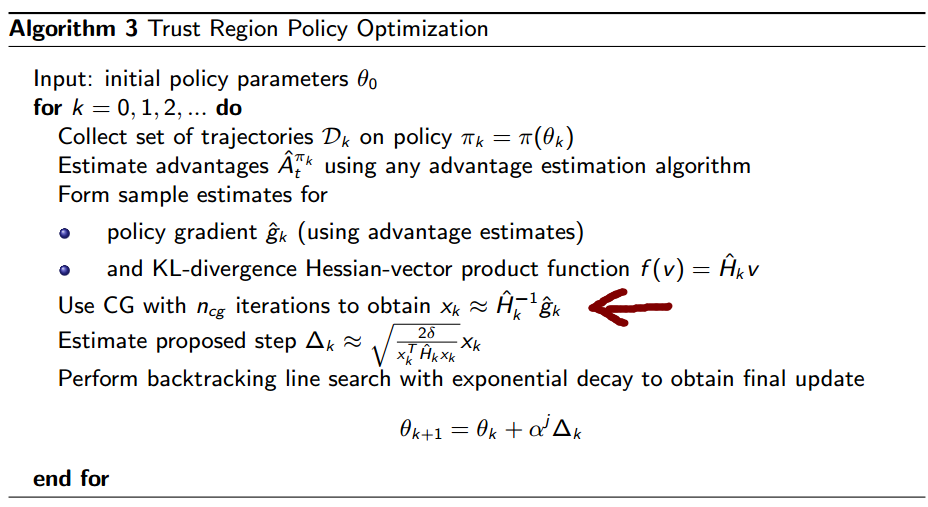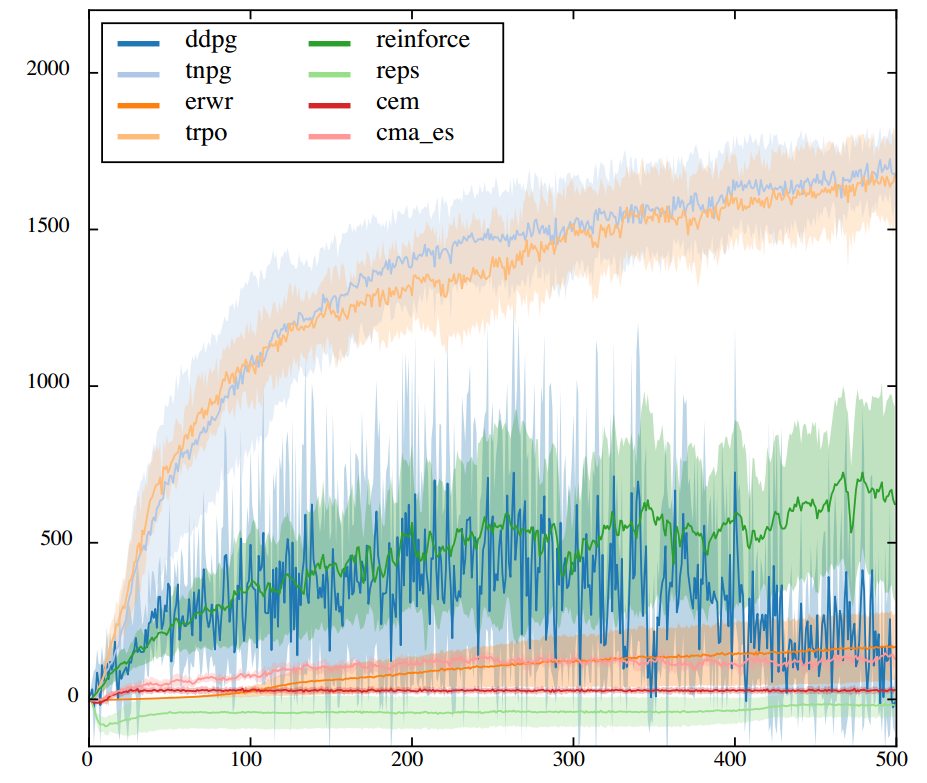### 13.3.3 Proximal Policy Optimization (PPO)

PPO算法可以进一步的优化计算量，PPO并不会去计算natural gradient，所以不会去计算$H^{-1}$。他会直接去计算一个带有截断效应的代理函数(clipped surrogate function)。虽然PPO没有啥理论依据，但是他的效果是很好的。PPO有两种实现：

1. Adaptive KL：他把原始的无约束优化问题中的$C$，变成了一个与迭代次数有关的书$\beta_{k}$。每次迭代会修正这个值
$\max_{\pi'} L_{\pi^{(k)}}(\pi' ) + \beta_k D_{KL}(\pi' \mid \mid \pi^{(k)})$
1. Clipped function：这时候的优化方程也是无约束的，用截断函数clip来替换了，$clip(A,B,C)$是说，如果A超过了最小值B，则等于B；超过最大值C，就取C，否则取A。另外$r_{t}(\theta)=\frac{\pi_\theta(a_{t} \mid s_{t})}{\pi_{\theta_{k}}(a_{t}\mid s_{t})}$，通常$\epsilon=0.2$

$\max_{\theta} L_{\theta_{k}} ^{Clip}(\theta)=E_{\tau \sim \pi_k} \left[\sum_{t=0}^T[\min(r_t(\theta)A_t^{\pi_k}, clip(r_t(\theta), 1-\epsilon, 1+\epsilon)A_t^{\pi_k})] \right]\\ \theta_{k+1} = \arg\max_{\theta} L_{\theta_{k}} ^{Clip}(\theta)$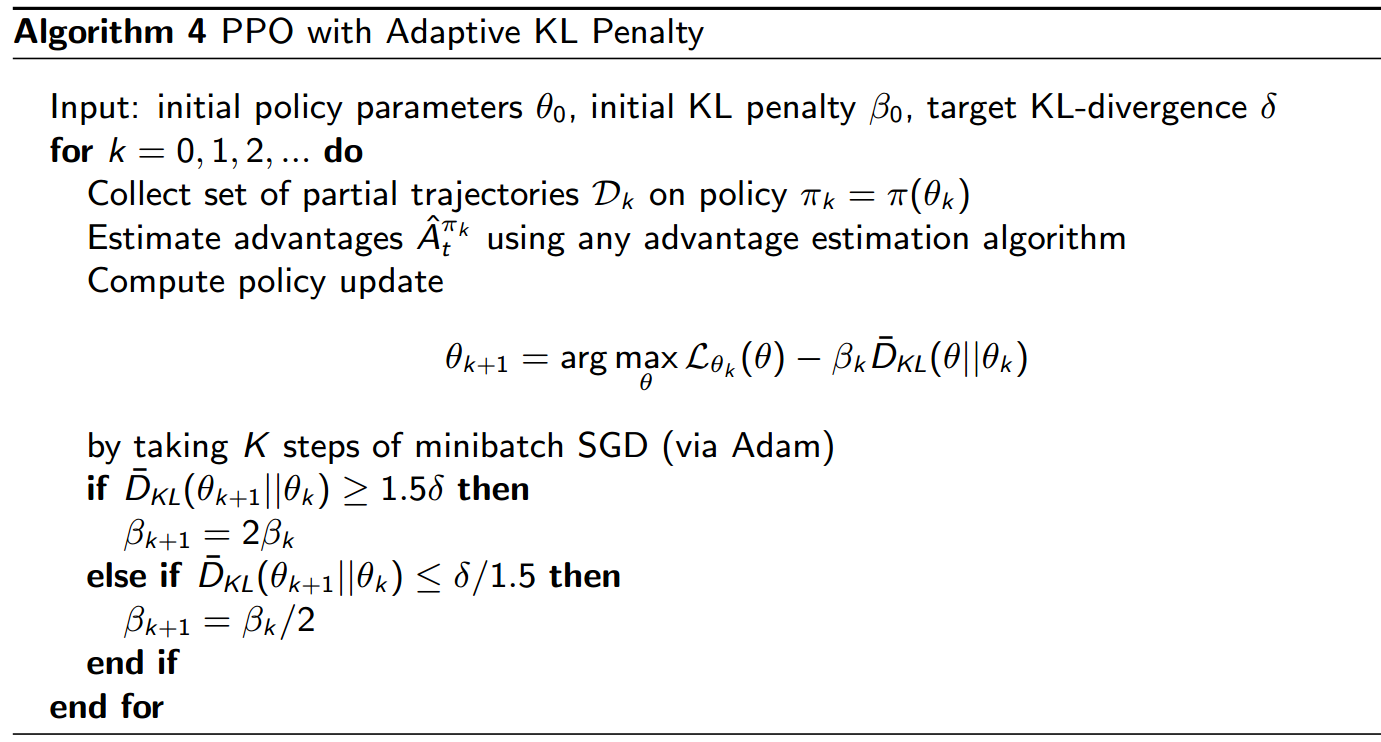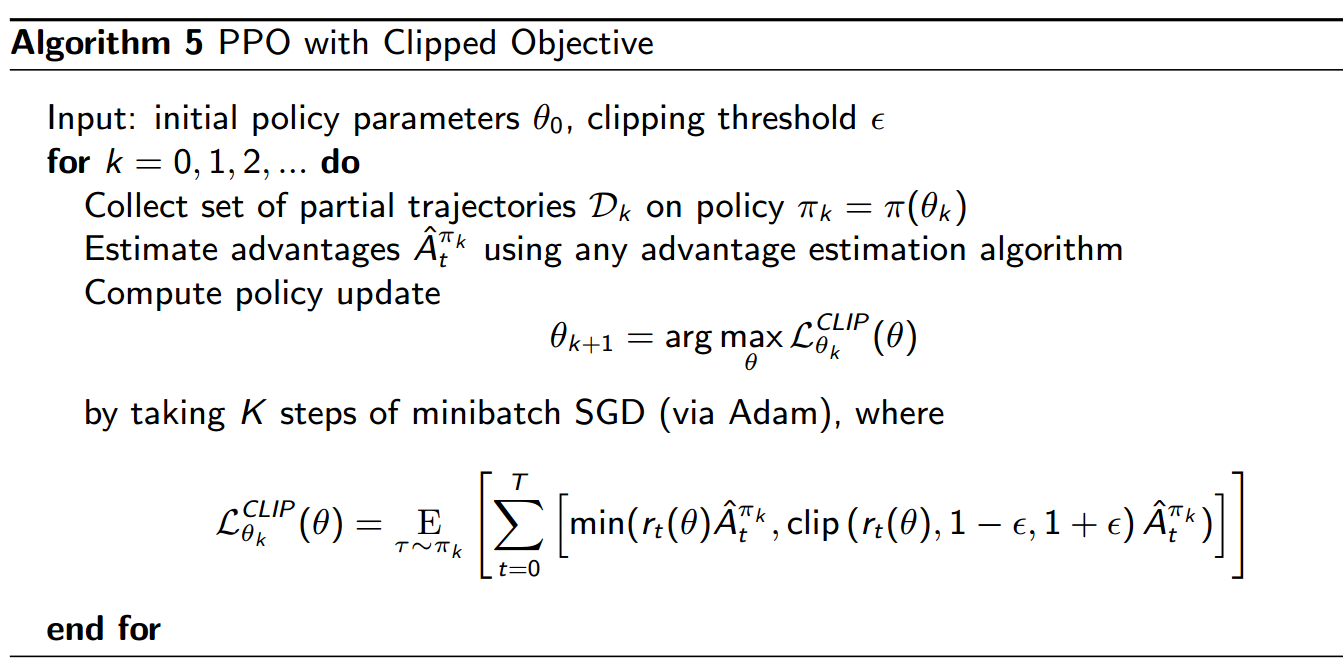# 14. Exploration and Exploitation

RL其中一个bug就在于搜索空间太大，难以找到全局最优解。这个问题可以等同于是探索（exploration）和利用（exploitation）之间的矛盾：到底是要根据已有的信息，做出决策；还是去探索未知，收集更多信息。探索未知，可能得不到一个好的（long-term或者short-term）reward，但是不去做，又没法知道。就好比，如果做action a1，可能得到10份，但是action a2的不确定性很高，它可能会带来5分到20分之间的一个reward，那么去不去做a2呢？这要求我们有一个好的探索策略，知道什么时候该去explore，什么时候该去exploit。

1. Naive/Greedy Exploration
2. Upper Confidence Bound
3. Posterior Sampling / Probability Matching
4. Information State Search
5. Information Gain based

## 14.1 Multi-Armed Bandits

Bandits问题就是有一台赌博机，每次pull arm的时候，他会给一个reward。Multi-Armed Bandits就是有多台这样的赌博机，每一台有一定的概率$P(r\mid a_{i})$给一个reward，但是玩家并不知道任何一台赌博机出现reward大小的概率分布，玩家需要每次选择一个机器，然后pull arm，得到rewardMulti-armed bandits可以看做是一个特殊的MDP，他没有状态空间，并且action space有m个动作，对应m个bandits。第$t$时刻，动作$a_{t}$表示去拉某一个机器的arm，每次动作之后会有一个反馈$r_{t}=R^{a_{t}}$。所以，我们的目标是最大化累计reward $\sum_{t}r_{t}$。这里没有discount，是因为Multi-armed bandits的episode长度为1，即，拉完一个arm之后，这个episode就结束了。

$V^\star = Q(a^\star)=\max_{a} Q(a)\\ Q(a)=E[r\mid a]$

$Regret=E\left[ \sum_{\tau}^t V^\star - Q(a_\tau)\right]$

$Regret=E\left[ \sum_{\tau}^t V^\star - Q(a_\tau)\right]=\sum_a E[N_t(a)]\triangle_a$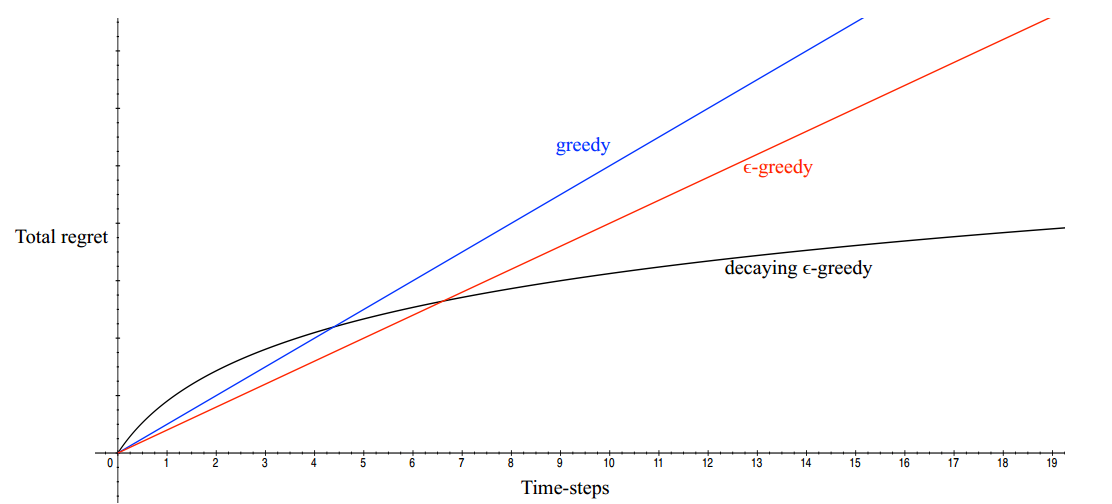Multi-armed bandits作为很多问题的基础，是因为它具有很好的理论基础。随着问题背景的复杂度增加，理论上也渐渐失去保证了，exploration的应用难度也渐渐加大。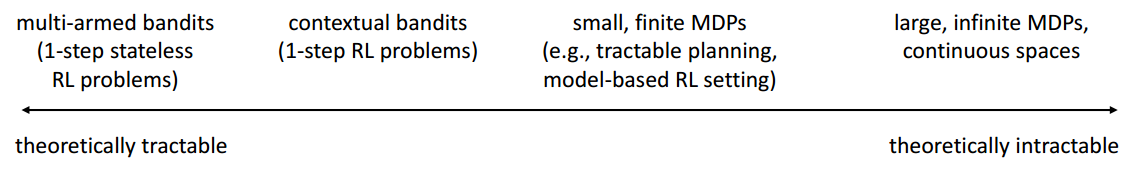## 14.2 Naive/Greedy Exploration

### 14.2.1 Greedy

$\widehat{Q}_t(a)=\frac{1}{N_t(a)} \sum_{t=1}^T r_t \mathbf{1}(a_t=a)$

### 14.2.2 $\epsilon-$greedy & Initialization

$\epsilon_t=\min(1, \frac{c\mid A \mid }{d^2t})\\ d=\min_{a \mid \triangle_a > 0} \triangle_i\\ c>0$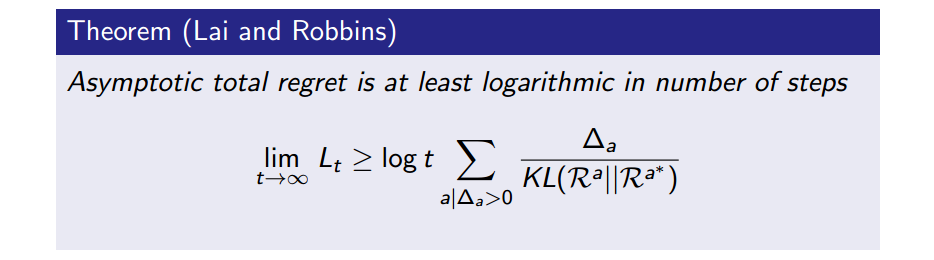## 14.3 Upper Confidence Bound

Upper Confidence Bound，简称UCB。这个方法在介绍MCTS的时候用提到了，他在选择action的时候，还可靠了action的不确定性（带来10分的action V.S. 可能带来5到20分的action）。如下图所示，通过很多次的实验，我们计算出三个机器给的reward分布。一号机$Q(a_{1})$的均值大概在$2.5$，并且方差很小，也就说，选择一号机很大可能他会给出2.5的reward。同理二号机是2，三号机1.5。这时候应该如何选择呢？如果选择1号，那么大概率是2.5分，但是从2号或者3号得到大于2.5分的reward的概率是不小的，尤其是3号，他的不确定性最大，得高分的可能性也最大。UCB提供了一个综合考虑的策略，他认为不确定性越大的action，越应该去探索，有可能那会是更好的action。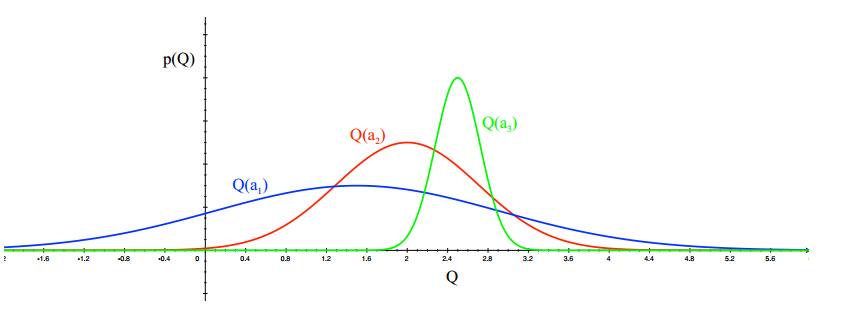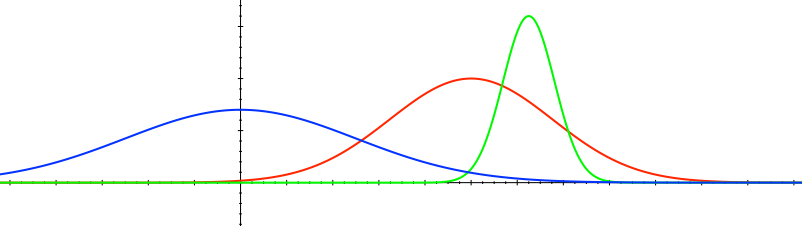$a_t=\arg\max_a \widehat{Q}_t(a) + U_t(a)$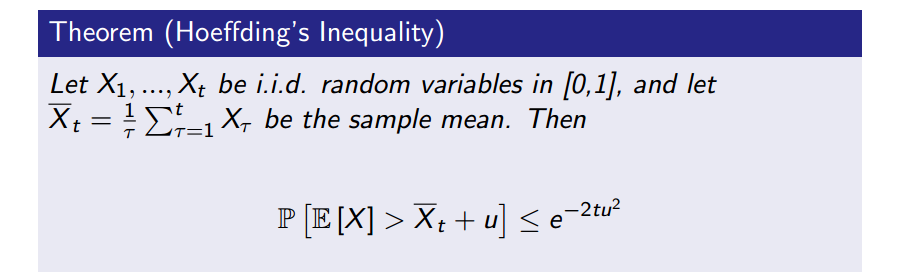UCB可以套用这个不等式来计算$U_{t}(a)$。套用下来就是

$P\left(Q_t(a)>\widehat{Q}_t(a)+U_t(a)\right) \leq e^{-2N_t(a)U_t(a)^2}$

$U_t(a)=\sqrt{\frac{-\log p}{2N_t(a)}}$

$U_t(a)=\sqrt{\frac{2\log t}{N_t(a)}}\\ a_t=\arg\max_a \widehat{Q}_t(a) + \sqrt{\frac{2\log t}{N_t(a)}}$

$U_t(a)=\sqrt{\frac{1}{N_t(a)}} \mbox{ 或者 } U_t(a)=\frac{1}{N_t(a)}$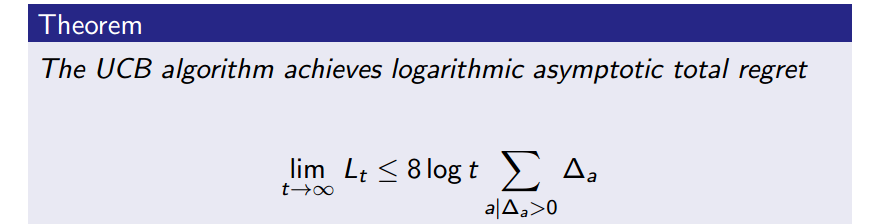## 14.4 Posterior Sampling / Probability Matching

Posterior Sampling是一种以概率选择的方法，它在历史实验的情况下，根据action是最优action的概率来进行选择

$\pi(a\mid h_t)=P(Q(a) > Q(a'), \forall a' \neq a \mid h_t)$

$a_t= \arg\max \mu_a + \frac{c \sigma_a}{\sqrt{N_t(a)}}$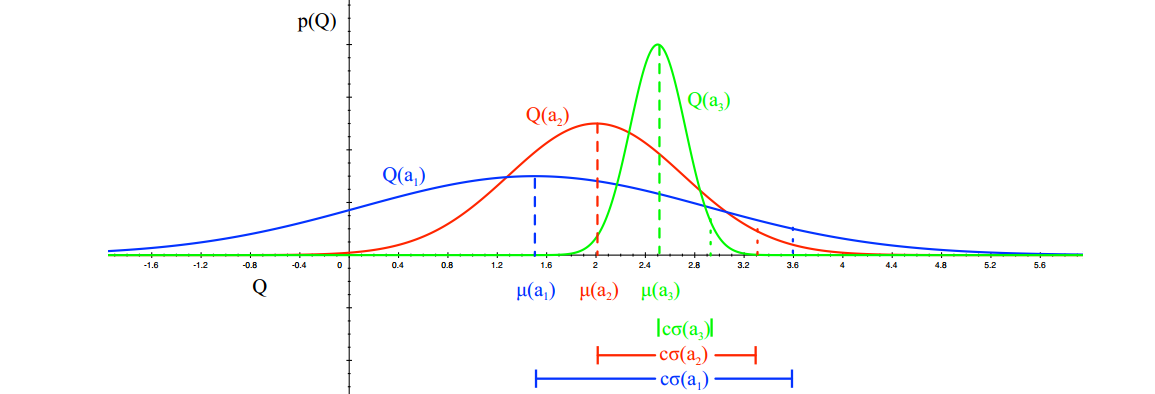$\pi(a\mid h_t)=P(Q(a) > Q(a'), \forall a' \neq a \mid h_t)\\ =E_{R \mid h_t} \left[ \mathbf{1}(a=\arg\max_a Q(a)) \right]$

1. 计算后验$P$（比如，上面的例子，我们算到了均值和方差的后验分布）
2. 从后验 $P$中采样出reward分布（从每一个bandit的后验采样出一个均值和方差，这样每个bandits上的reward分布也就确定了）
3. 选择动作 $a_{t}=\arg\max_{a} Q(a)$（比如采用UCB的方法来计算）
4. 根据选择的结果，更新后验分布$P$

Information State Search是一个新的视角。他把bandits转变成了连续决策的过程。原始bandits是没有state的，这里给它加了一个Information state。所谓的information state $s$是指带一个历史信息的总和，即$s_{t}=f(h_{t})$。比如当$t=0$的时候，$s_{0}$表示一次都没有pull；如果$t=1$的时候，以一定的选择概率$P$，拉了一号，那么$s_{1}$就表示一号拉了一次，其他0次。所以这个又变成了一个MDP了：${S,A,P,R,\gamma}$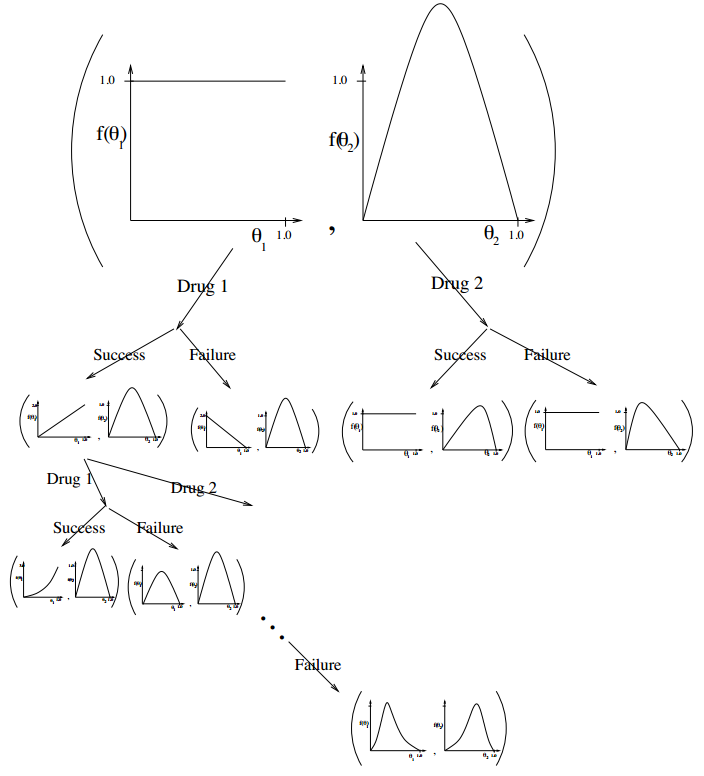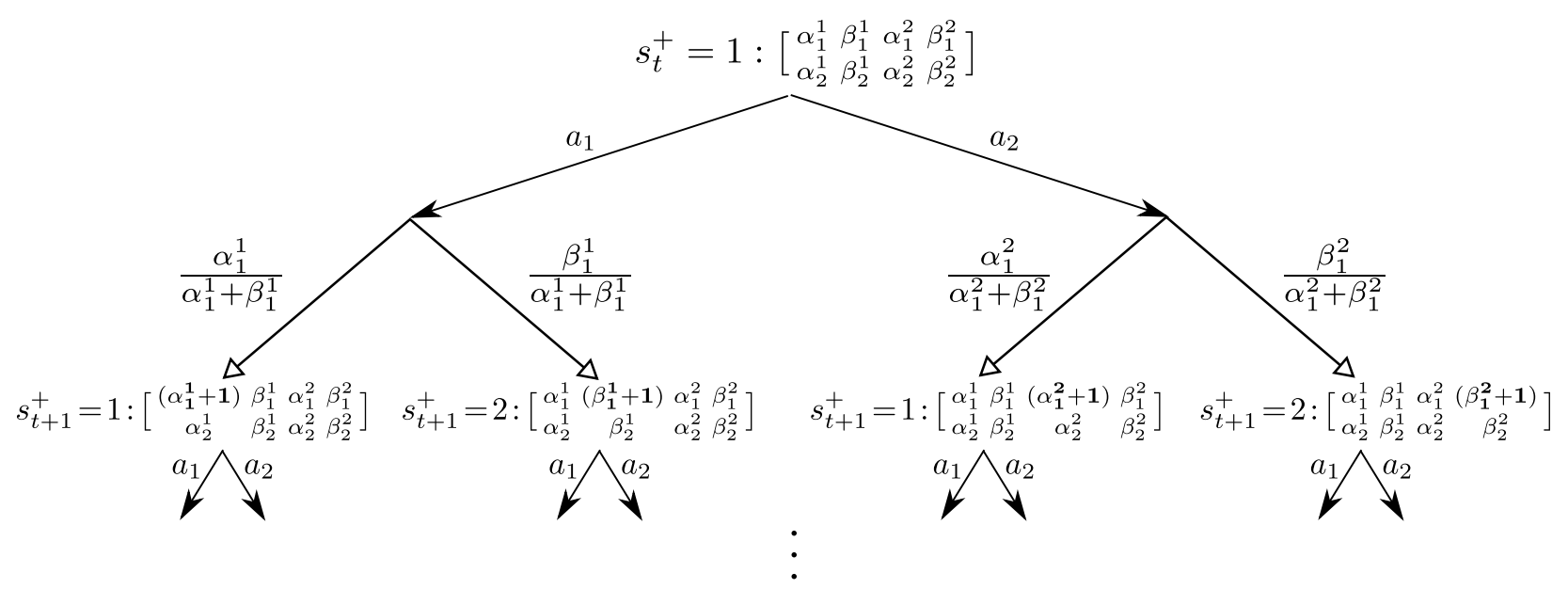## 14.6 Information Gain

$IG(z,y\mid a) = E_y\left[ H(P(z))-H(P(z)\mid y) \mid a \right]$

$\arg\min_a \frac{\triangle_t(a)^2}{IG(\theta_a, r_a\mid a)}$

## 14.7 RL in Action

Multi-armed bandits相比于RL来说，问题简单了不少。本部分主要就RL的exploration方法做一个简述。总的来说，上面提到的方法在RL中都可以应用。比如基于Naive method或者UCB的方法，但首先要解决的问题是怎么对某个状态的访问次数进行计数。这个问题在连续action space下，用常规的方法是“数”不过来的。

### 14.7.1 How to count $N_{t}(a)$ or $N_{t}(s)$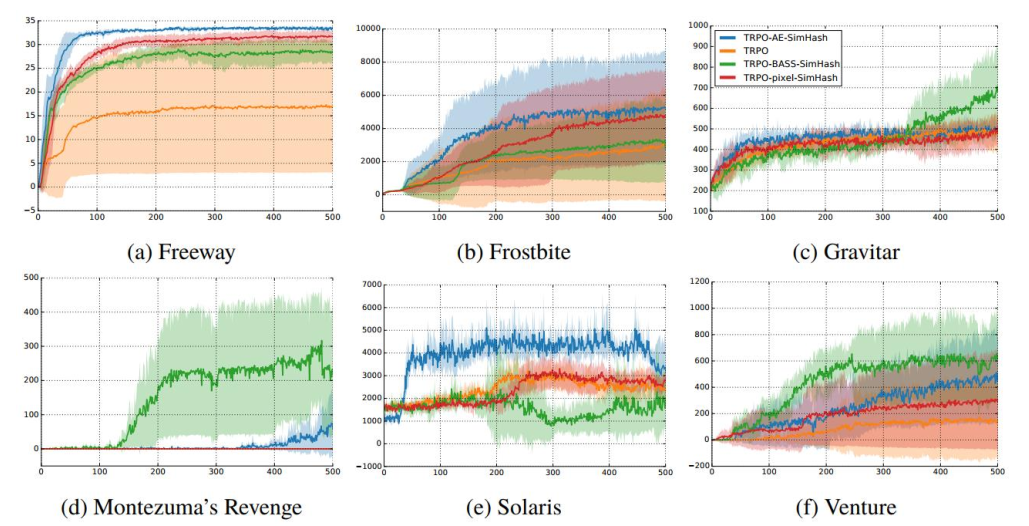$P_{\theta}(s)=\frac{N(s)}{n}\\ P_{\theta'}(s)=\frac{N(s)+1}{n+1}$

$N(s)=nP_\theta(s) = \frac{1-P_{\theta'}(s)}{P_{\theta'}(s)-P_\theta(s)}P_\theta(s)^2$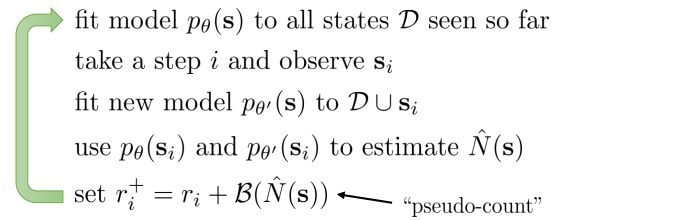EX2: Exploration with Exemplar Models for Deep Reinforcement Learning

$P_\theta(s) = \frac{1-D_s(s)}{D_s(s)}$

### 14.7.2 UCB in RL

UCB的方法基本和所有的RL算法都很兼容，这时候只需要将reward设置为$r(s,a)+ e \sqrt{\frac{2\log t}{N(a)} }$。这里$e$是两个部分的融合权重，怎么设置就需要调参咯

### 14.7.3 Posterior sampling in RL

posterior sample与RL的结合方式是通过Off-policy。比如在Q-learning，我们其实并不关心要基于那个Q函数来求max，选action。所以可以吧这个Q当做是一个从posterior 中采样出来的样本。这么做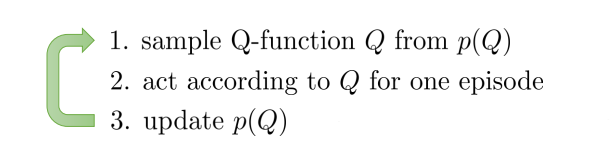### 14.7.4 Naive Method in RL

1. 使用$\epsilon-$greedy的方法，同时结合那个特殊的初始化方法，然后就可以正常的跑Q-learning, sarsa, MC等等model-free RL方法。
2. 或者采用model-based方法，在学习reward和transition的时候，初始化每个状态的reward都最大。然后随着学习的进行，用table lookup的方法统计和学习真正的reward。这个方法和有特殊初始化的$\epsilon-$greedy方法如出一辙，该算法叫RMax